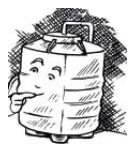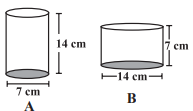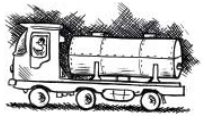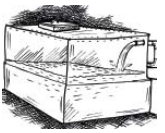# Class 8 maths chapter 11 mensuration solution Ex 11.4

In this page we have Class 8 maths chapter 11 mensuration solution Ex 11.4.This exercise has questions about Volume of cube, cuboid and Cylinder.It has also questions about Capacity. Hope you like them and do not forget to like , social share and comment at the end of the page.

## Class 8 maths chapter 11 mensuration solution Ex 11.4

Question 1
Given a cylindrical tank, in which situation will you find surface area and in which situation volume.
(a) To find how much it can hold.
(b) Number of cement bags required to plaster it.
(c) To find the number of smaller tanks that can be filled with water from it.(a) We need to calculate the volume to find the capacity
(b) As plastering will cover the surface so we need surface area to know this
(c) Volume will give the capacity and that can be compared with capacity of smaller tanks

Question 2
Diameter of cylinder A is 7 cm, and the height is 14 cm. Diameter of cylinder B is 14 cm and height is 7 cm. Without doing any calculations can you suggest whose volume is greater? Verify it by finding the volume of both the cylinders. Check whether the cylinder with greater volume also has greater surface area?As cylinder A’s radius is half of radius of cylinder B so its volume will be lesser than that of cylinder B. Although Cylinder B’s height is half of height of cylinder A but as you know while calculating the volume we need to square the radius so halving the radius has a greater impact than halving the height.
Volume = πr2H
Volume  of Cylinder A= (22/7)(7/2)2 14=539
Volume  of Cylinder B= (22/7)(7)2 7=1078
So Volume Of Cylinder B is greater than Volume of cylinder A
Total Surface Area of Cylinder is given by
= 2πr(r+H)
Total Surface Area of Cylinder A= 385 cm2
Total Surface Area of Cylinder B= 616 cm2
So Cylinder will greatest volume has greater surface area

Question 3
Find the height of a cuboid whose base area is 180 cm2 and volume is 900 cm3?
Volume of cuboid   is given by
=LBH
= Base Area x Height
Now given here
V=900cm3 and Base Area=180cm2
So
900=180H
Or H=5cm

Question 4
A cuboid is of dimensions 60 cm × 54 cm × 30 cm. How many small cubes with side 6 cm can be placed in the given cuboid?
Number of small cubes will be given by
= (Volume of Big Cube)/(Volume  of small cube)
=60×54×30/6×6×6
=450

Question 5
Find the height of the cylinder whose volume is 1.54 m³ and diameter of the base is 140 cm ?
Volume of cylinder is given by
=πr2h
Here V=1.54 m3
r=  70 cm=.7 m
So
(22/7) (.7)2 H= 1.54
H=1 m

Question 6
A milk tank is in the form of cylinder whose radius is 1.5 m and length is 7 m. Find the quantity of milk in litres that can be stored in the tank?Volume of Milk Tank= πr2H
=49.5 m3
Now As we know, 1 cubic metre = 1000 litres
So, 49.5 m3 = 49500 litres

Question 7
If each edge of a cube is doubled,
(i) how many times will its surface area increase?
(ii) how many times will its volume increase?
Let a be the side of the cube
Surface Area of Cube= 3a2
Volume of Cube=a3
(i) So Whenever sides are doubled in any structure then area becomes 4 times the original structure
(ii) Volume becomes 8 times of the original volume if sides are doubled in any structure

Question 8
Water is pouring into a cuboidal reservoir at the rate of 60 liters per minute. If the volume of reservoir is 108 m³, find the number of hours it will take to fill the reservoir.108 cubic meter = 108000 liter
So, time = Volume Rate per minute
=108000/60 minutes
= 108000/(60 ×60)
=30 hours

## Summary

1. Class 8 maths chapter 11 mensuration solution Ex 11.4 has been prepared by Expert with utmost care. If you find any mistake.Please do provide feedback on mail. You can download the solutions as PDF in the below Link also
2. This chapter 11 has total 4 Exercise 11.1 ,11.2 ,11.3 and 11.4. This is the Fourth and last exercise in the chapter.You can explore previous exercise of this chapter by clicking the link below